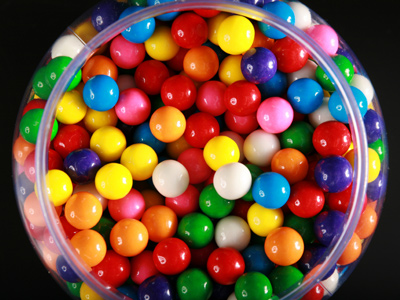In a jar of 120 candies, one in every six candies is orange flavored.

# Numbers - Fractions - Multiplication and Division

This Math quiz is called 'Numbers - Fractions - Multiplication and Division' and it has been written by teachers to help you if you are studying the subject at middle school. Playing educational quizzes is a fabulous way to learn if you are in the 6th, 7th or 8th grade - aged 11 to 14.

It costs only \$12.50 per month to play this quiz and over 3,500 others that help you with your school work. You can subscribe on the page at Join Us

Multiplication (or 'times') and division of fractions is an important subject in Math and you will come across fractions not only in your lessons but in everyday life too.

Practice your numbers skills in the following quiz on multiplication and division of fractions.

1.
14,000 fans attended the soccer match yesterday. 6/7 of them wore scarves. How many were without scarves?
2,000
4,000
8,000
10,000
6/7 of 14,000 = 12,000, therefore 2,000 were without scarves
2.
A piece of copper wire is 723m long. What is the total length of three of these wires?
2238
23
2413
26
3.
What is 214 x 215?
2310
3510
41920
512
4.
What is 234 ÷ 418?
1/3
1/2
2/3
3/4
5.
What is 914 ÷ 112?
1/7
2/7
3/7
5/7
To divide by a fraction, invert and multiply
6.
Daniel is making pancakes. Each pancake uses 18 of a liter of batter. How much batter is used if Daniel makes 20 pancakes?
212 liters
234 liters
313 liters
413 liters
I'll have lemon and sugar on mine please!
7.
What is 310 ÷ 625?
1
114
112
134
To divide by a fraction, invert and multiply: 3/10 ÷ 6/25 = 3/10 x 25/6. Use this method whenever you have to divide by fractions
8.
What is 1/9 x 3/5?
1/12
1/14
1/15
1/18
9.
What is 3/8 of £48.00?
£6.00
£10.00
£14.00
£18.00
Rewrite the question as 3/8 x 48. 48 / 8 = 6, 6 x 3 = 18, therefore the answer is £18.00
10.
In a jar of 120 candies, one in every six candies is orange flavored. If half the orange candies are eaten, what fraction of the candies is left?
56
34
1112
910
16 of 120 is 20, so 10 are eaten, leaving 110. 110120 = 1112
Author:  Frank Evans